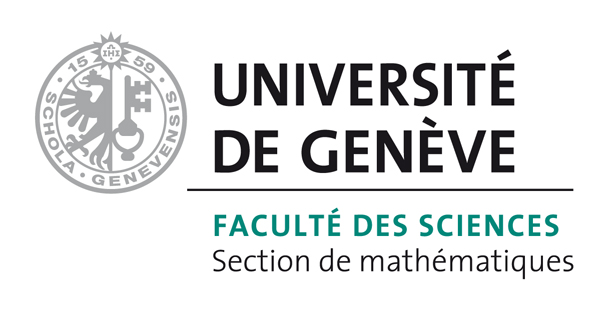# Bart Vandereycken## Automatic rational approximation and linearization of nonlinear eigenvalue problems

by , , ,

#### Abstract:

We present a method for solving nonlinear eigenvalue problems (NEPs) using rational approximation. The method uses the Antoulas\textendash Anderson algorithm (AAA) of Nakatsukasa, Sète and Trefethen to approximate the NEP via a rational eigenvalue problem. A set-valued variant of the AAA algorithm is also presented for building low-degree rational approximations of NEPs with a large number of nonlinear functions. The rational approximation is embedded in the state-space representation of a rational polynomial by Su and Bai. This procedure perfectly fits the framework of the compact rational Krylov methods (CORK and TS-CORK), allowing solve large-scale NEPs to be efficiently solved. One advantage of our method, compared to related techniques such as NLEIGS and infinite Arnoldi, is that it automatically selects the poles and zeros of the rational approximations. Numerical examples show that the presented framework is competitive with NLEIGS and usually produces smaller linearizations with the same accuracy but with less effort for the user.

#### Reference:

P. Lietaert, K. Meerbergen, J. Pérez, B. Vandereycken, "Automatic rational approximation and linearization of nonlinear eigenvalue problems", In IMA Journal of Numerical Analysis, no. draa098, 2021.

#### Bibtex Entry:

@article{Lietaert_MPV:2021,
title = {Automatic rational approximation and linearization of nonlinear eigenvalue problems},
author = {Lietaert, P. and Meerbergen, K. and P{\'e}rez, J. and Vandereycken, B.},
year = {2021},
month = feb,
issn = {0272-4979},
doi = {10.1093/imanum/draa098},
abstract = {We present a method for solving nonlinear eigenvalue problems (NEPs) using rational approximation. The method uses the Antoulas\textendash Anderson algorithm (AAA) of Nakatsukasa, S\ete and Trefethen to approximate the NEP via a rational eigenvalue problem. A set-valued variant of the AAA algorithm is also presented for building low-degree rational approximations of NEPs with a large number of nonlinear functions. The rational approximation is embedded in the state-space representation of a rational polynomial by Su and Bai. This procedure perfectly fits the framework of the compact rational Krylov methods (CORK and TS-CORK), allowing solve large-scale NEPs to be efficiently solved. One advantage of our method, compared to related techniques such as NLEIGS and infinite Arnoldi, is that it automatically selects the poles and zeros of the rational approximations. Numerical examples show that the presented framework is competitive with NLEIGS and usually produces smaller linearizations with the same accuracy but with less effort for the user.},
journal = {IMA Journal of Numerical Analysis},
number = {draa098},
Pdf = {http://www.unige.ch/math/vandereycken/papers/preprint_Lietaert_MPV_2020.pdf}
}`